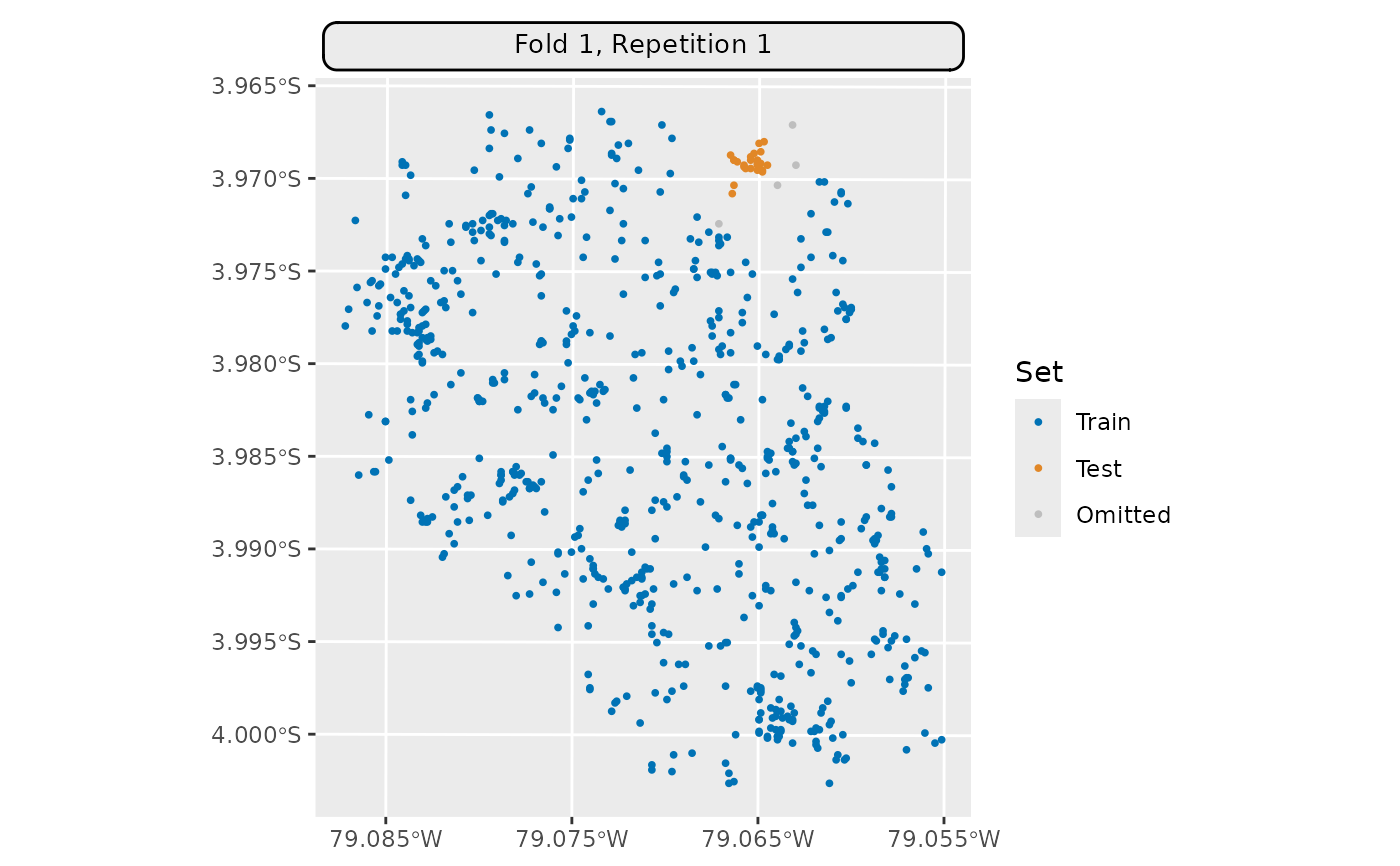Generic S3 plot() and autoplot() (ggplot2) methods to visualize mlr3 spatiotemporal resampling objects.

## Usage

# S3 method for ResamplingSpCVDisc
autoplot(
object,
fold_id = NULL,
plot_as_grid = TRUE,
train_color = "#0072B5",
test_color = "#E18727",
repeats_id = NULL,
show_omitted = FALSE,
sample_fold_n = NULL,
...
)

# S3 method for ResamplingRepeatedSpCVDisc
autoplot(
object,
fold_id = NULL,
repeats_id = 1,
plot_as_grid = TRUE,
train_color = "#0072B5",
test_color = "#E18727",
show_omitted = FALSE,
sample_fold_n = NULL,
...
)

# S3 method for ResamplingSpCVDisc
plot(x, ...)

# S3 method for ResamplingRepeatedSpCVDisc
plot(x, ...)

## Arguments

object

[Resampling]
mlr3 spatial resampling object of class ResamplingSpCVBlock or ResamplingRepeatedSpCVBlock.

[TaskClassifST]/[TaskRegrST]

fold_id

[numeric]
Fold IDs to plot.

plot_as_grid

[logical(1)]
Should a gridded plot using via patchwork be created? If FALSE a list with of ggplot2 objects is returned. Only applies if a numeric vector is passed to argument fold_id.

train_color

[character(1)]
The color to use for the training set observations.

test_color

[character(1)]
The color to use for the test set observations.

repeats_id

[numeric]
Repetition ID to plot.

show_omitted

[logical]
Whether to show points not used in train or test set for the current fold.

sample_fold_n

[integer]
Number of points in a random sample stratified over partitions. This argument aims to keep file sizes of resulting plots reasonable and reduce overplotting in dense datasets.

...

Passed to geom_sf(). Helpful for adjusting point sizes and shapes.

x

[Resampling]
mlr3 spatial resampling object. One of class ResamplingSpCVBuffer, ResamplingSpCVBlock, ResamplingSpCVCoords, ResamplingSpCVEnv.

## Details

This method requires to set argument fold_id and no plot containing all partitions can be created. This is because the method does not make use of all observations but only a subset of them (many observations are left out). Hence, train and test sets of one fold are not re-used in other folds as in other methods and plotting these without a train/test indicator would not make sense.

## 2D vs 3D plotting

This method has both a 2D and a 3D plotting method. The 2D method returns a ggplot with x and y axes representing the spatial coordinates. The 3D method uses plotly to create an interactive 3D plot. Set plot3D = TRUE to use the 3D method.

Note that spatiotemporal datasets usually suffer from overplotting in 2D mode.

• mlr3book chapter on "Spatiotemporal Visualization"

• Vignette Spatiotemporal Visualization.

• autoplot.ResamplingSpCVBlock()

• autoplot.ResamplingSpCVBuffer()

• autoplot.ResamplingSpCVCoords()

• autoplot.ResamplingSpCVTiles()

• autoplot.ResamplingSpCVEnv()

• autoplot.ResamplingCV()

• autoplot.ResamplingSptCVCluto()

## Examples

# \donttest{
if (mlr3misc::require_namespaces("sf", quietly = TRUE)) {
library(mlr3)
library(mlr3spatiotempcv)